Mathematics
Easy

Question

# In an inverse variation, x = 6 and y =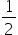. What is the equation that represents the inverse variation?

## y =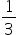xy =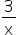y = -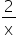y =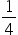xHint:

## The correct answer is: y =### Here, we are given that in an inverse variation, x = 6 and y = 1/2.We have to find the relation between x and y.We have, y =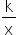=>= k/6=> k = 3.Equation: y =.Hence, the correct option is B.

We can also take the relation as xy = k instead of y = k/x, where k is a constant.

### Related Questions to study#### With Turito Foundation.#### Get an Expert Advice From Turito.